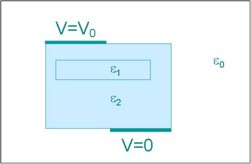# Electro Static: presentation and typical example

## Presentation

The Electro Static application allows the user to study the electric charges systems at equilibrium.

## Use

Examples of Electro Static applications are high voltage devices, spark-gaps, insulators, etc.

## Typical example

A typical example of problem treated as an Electro Static application is presented in the figure below.## Principal results

The principal results that can be obtained with the Electro Static application are:

• the space distribution of the electric potential (V), of the electric field intensity (E), of the electric flux density (D) and of the volume density of electrostatic energy (dWe)
• the electrostatic energy (We) in a region or in the whole study domain, the electrostatic force (Fe) or the electrostatic torque (Γe) on a region
• the electric charge carried by the surface of the conductors (q)

By means of these quantities, it is possible to compute other useful quantities, for example capacitance (or the matrix of capacitance), etc.

## Example

An example of an electrostatic problem is shown in the figure below.